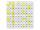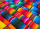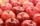# Numbers - math word problems

#### Number of problems found: 2341

• Unknown numberI think the number. I'll reduce it to its one-third. The result is then increased by one-third, and I get the number 12.
• I thinkI think a number. When I multiply it by five, and after that I subtract 477, I get the same number as if I multiplied it twice. What number do I think?
• The expressionWhat is the value of the expression ((62+60))/(23)
• NumberI think the number. If I add to its third seven I get same as when to its quarter add 8. Which is the number?
• Watching TVOne evening 2/3 of students watch TV. Of those students, 3/8 watched a reality show. Of the students that watched the show, 1/4 of them recorded it. What fraction of the students watched and recorded reality tv.
• Equation with fractionsWhat is another way to write the equation 7/8 x + three-fourths = negative 6?
• The sum 9The sum of two integers is 4. Their difference is 8. What are this two integers?
• Primes 2Which prime numbers is number 2025 divisible?
• PearsThere were pears in the basket, I took two-fifths of them, and left six in the basket. How many pears did I take?
• LCD 2The least common denominator of 2/5, 1/2, and 3/4
• Fractions and mixed numerals(a) Convert the following mixed numbers to improper fractions. i. 3 5/8 ii. 7 7/6 (b) Convert the following improper fraction to a mixed number. i. 13/4 ii. 78/5 (c) Simplify these fractions to their lowest terms. i. 36/42 ii. 27/45 2. evaluate the follow
• The sum 16The sum of A and B is 36. The difference between A & B is 8. find the larger number of A.
• Two numbersThe sum of the two numbers is 1. Find both numbers if you know that half of the first is equal to one-seventh of the second number.
• UN 1If we add to an unknown number his quarter, we get 210. Identify unknown number.
• Hannah and EmilyHannah and Emily need to use  4 1/4  feet of ribbon to finish an art project. Hannah has 2 3/4 feet of ribbon and Emily has 1 3/4 feet of ribbon. How much ribbon will they have left over after they finish their art project?
• Distributive propertyVerify the distributive property a×(b+c)=(a×b)+(a*c) for the rational number a=5/8, b=7/4 and c=2/3
• Simple equation 324 = n • 27, solve for n
• Trees3/5 trees are apples, cherries are 1/3. 5 trees are pear. How many is the total number of trees?
• Apples 2How many minimum apples are in the cart, if possible is completely divided into packages of 6, 14 and 21 apples?
• Find unknown 2Find unknown denominator: 2/3 -5/? = 1/4

Do you have an exciting math question or word problem that you can't solve? Ask a question or post a math problem, and we can try to solve it.

We will send a solution to your e-mail address. Solved examples are also published here. Please enter the e-mail correctly and check whether you don't have a full mailbox.